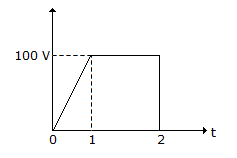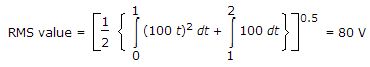# Electronics and Communication Engineering - Networks Analysis and Synthesis

1.
In a parallel resonant circuit, the circuit current at resonance is maximum.
True
False
Explanation:

In parallel resonance current is minimum.

2.
The rms value of wave in figure isExplanation:3.
An RLC series circuit is underdamped. To make it overdamped, the value of R
has to be increased
has to be decreased
has to be increased to infinity
has to be reduced to zero
Explanation:

For overdamping R is more.

4.
Henry is equivalent to
Volts/Ampere
Weber/Volt
Weber/Ampere
Weber/Ampere2
Explanation:

Inductance is flux per ampere.

5.
In a minimum function
the degree of numerator and denominator are equal
the degree of numerator and denominator are unequal
the degree of numerator is one more than degree of denominator
the degree of numerator is one less than degree of denominator